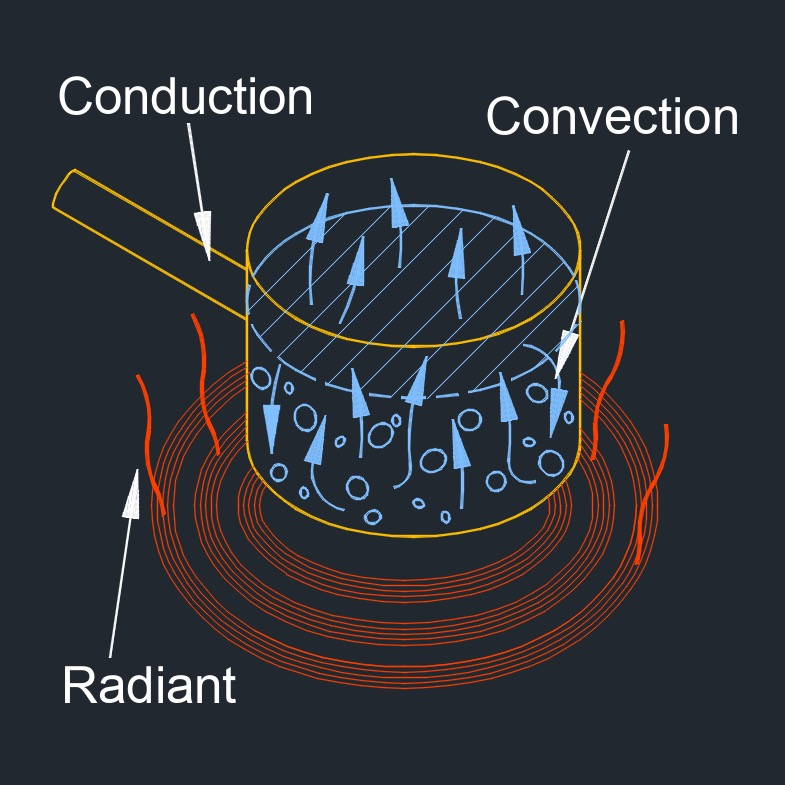# Heat Transfer Coefficient

on . Posted in ThermodynamicsHeat transfer coefficient, abbreviated as h, also called film coefficient or film effectiveness, is the convective heat transfer between a solid surface and the fluid around it.

## Heat Transfer Coefficient formula

$$\large{ h = \frac { Q" }{\Delta T} }$$
Symbol English Metric
$$\large{ h }$$ = heat transfer coefficient $$\large{\frac{Btu}{hr-ft^2-F}}$$  $$\large{\frac{W}{m^2-K}}$$
$$\large{ Q" }$$ = heat flux $$\large{\frac{Btu}{hr-ft^2}}$$  $$\large{\frac{W}{m^2}}$$
$$\large{ \Delta T }$$ = temperature differential between the solid surface and surrounding area  $$\large{F}$$ $$\large{K}$$